Gear

Two gears, fit into each other, has transfer 2:3. Centres of gears are spaced 82 cm. What are the radii of the gears?

Result

r1 =  49.2 cm
r2 =  32.8 cm

Solution:Leave us a comment of this math problem and its solution (i.e. if it is still somewhat unclear...):Be the first to comment!To solve this verbal math problem are needed these knowledge from mathematics:

Do you have a linear equation or system of equations and looking for its solution? Or do you have quadratic equation?

Next similar math problems:

1. MushroomsEva and Jane collected 114 mushrooms together. Eve found twice as much as Jane. How many mushrooms found each of them?
2. AgesJohn, Teresa, Daniel and Paul have summary 56 years. Their ages are in a ratio of 1:2:5:6. Determine how many years have each of them.
3. Factory and divisionsThe factory consists of three auxiliary divisions total 2,406 employees. The second division has 76 employees less than 1st division and 3rd division has 212 employees more than the 2nd. How many employees has each division?
4. TestingStudents of high school have 10 points for each good solved task. The wrong answer is deducted by 5 points. After solving 20 tasks, student Michael had 80 points. How many tasks did he solve correctly and how many wrong?
5. Theorem proveWe want to prove the sentence: If the natural number n is divisible by six, then n is divisible by three. From what assumption we started?
6. Hotel roomsIn the 45 rooms, there were 169 guests, some rooms were three-bedrooms and some five-bedrooms. How many rooms were?
7. Mom and daughterMother is 39 years old. Her daughter is 15 years. For many years will mother be four times older than the daughter?
8. Simple equation 8Solve the following equation: 36=-(1+7x)-6(-7-x)
9. Borrowed book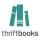Jane must as soon as possible return a borrowed book. She figured that when she read 15 pages a day return book in time. Then she read 18 pages a day and then return the book one day before. How many pages should have a book?
10. Buttons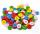Shirt has 6 buttons, blouse has 4 buttons. All buttons are 176. Shirts and blouses are together 36. How many are the shirts and blouses?
11. Equation 29Solve next equation: 2 ( 2x + 3 ) = 8 ( 1 - x) -5 ( x -2 )
12. Men, women and childrenOn the trip went men, women and children in the ratio 2:3:5 by bus. Children pay 60 crowns and adults 150. How many women were on the bus when a bus was paid 4,200 crowns?
13. Farm2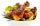The farm bred turkeys, geese, hens and chickens. Everything is 400. Turkeys and geese a hen are 150. No hen has more than one chicken, but some hens has no chick. Half of them is also a quarter of the chickens. When the turkey subtracting 15, we get 75. Ho
14. Magic numberThe number 135 split to two addends so that one addend was 30 greater than 2/5 the addend.
15. Mushrooms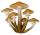For five days, we have collected 410 mushrooms. Interestingly every day we have collected 10 mushrooms more than the preceding day. How many mushrooms we have collected during 4th day?
16. Chicken and rabbits (classic)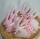Grandmother breeding chickens and rabbits. It found that the yard has 35 heads and 108 legs. How many chickens and rabbits breeding in the yard?
17. Substitutionsolve equations by substitution: x+y= 11 y=5x-25